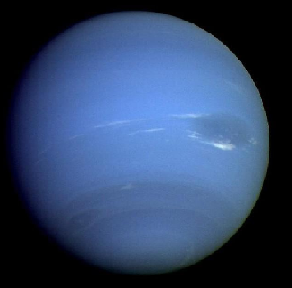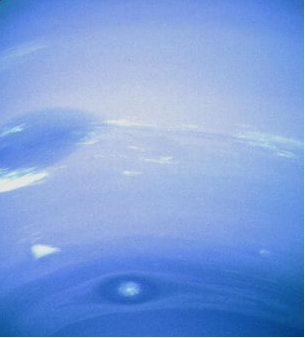﻿ Weight On Neptune Calculator ﻿# Weight on Neptune Calculator### How Much Would You Or Any Object Weigh On Neptune?Enter Weight: Any unit grams (g) kilograms (kg) pounds (lbs)

This Weight on Neptune Conversion Calculator calculates your weight or the weight that any object would be if on Neptune.

To use this calculator, a user just enters his or her weight or the object's weight and clicks the 'Calculate' button, and the weight will automatically be computed and shown below. The weight can be entered in any units and the resultant answer will be in the same units which the user has input. Thus, for example, if a user enters 150lbs, the resultant answer of the weight in Neptune would be 159.63lbs. The units will always match. Just for the sake of clarity, a user can select the units he wants the answer to appear in, whether grams, kilograms, or pounds, and that unit will show in the answer.

This calculator calculates the weight on Neptune from the weight of the object on earth. Being that it takes the weight of an object on earth and converts it to the weight on Neptune, the formula is Weight on Neptune= (Weight on Earth/9.81m/s2) * 11.15m/s2. To find the weight on Neptune, we divide the weight on earth by the earth's force of gravity, which is 9.81m/s2. This calculates the mass of the object. Once we have the object's mass, we can find the weight by multiplying it by the gravitational force, which it is subject to. Being that Neptune has a gravitational force of 11.15m/s2, we multiply the object's mass by this quanitity to calculate an object's weight on Neptune.

So an object or person on Neptune would weigh 113.66% of its weight on earth. Therefore, a person would be heavier on Neptune than on earth. Conversely, a person is 85.7% lighter on earth than on Neptune.

What causes the differences in weight between the various planets? The answer is the gravitational force which a planet is subject to. Earth has less mass than Neptune. Therefore, the sun pulls on the earth with lesser force, because of the lesser mass. You probably are familiar that gravity is a downward force. It pushes you back to the Earth's surface. This is why when you jump, you come back down. Being that the earth has lesser gravitational force, it pulls object downward with lesser force. This translates into lesser weight.

If you want to calculate the weight of all of the planets simultaneously, see our Weight on Planets Calculator.

Related Resources

﻿